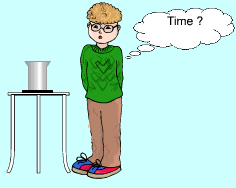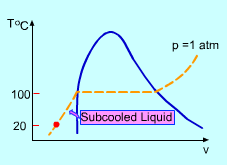Ch 2. Pure Substances Multimedia Engineering Thermodynamics Phase PropertyDiagrams PropertyTables IdealGas
 Chapter 1. Basics 2. Pure Substances 3. First Law 4. Energy Analysis 5. Second Law 6. Entropy 7. Exergy Analysis 8. Gas Power Cyc 9. Brayton Cycle 10. Rankine Cycle Appendix Basic Math Units Thermo Tables eBooks Dynamics Fluids Math Mechanics Statics Thermodynamics Author(s): Meirong Huang Kurt Gramoll ©Kurt GramollTHERMODYNAMICS - CASE STUDY IntroductionPhase Change Experiment A student is working on his thermodynamics experiment about phase change. He boils room temperature water using a pan placed on the top of a electric heater at room pressure. A thermometer is used to measure the temperature of the substance in the pan. Known: The mass of the water in the pan is m = 1.0 kg The power (energy per unit time) of the electric heater is= 2.0 KW The temperature of the room is Troom = 20 oC and the pressure is P = 1 atm The specific heat of water is c = 4.184 kJ/(kg-K) The latent heat of vaporization of water is L = 2257.0 kJ/kg QuestionsPhase Change Process on T-v Diagram The student needs to record the temperature over a period of time. The records should include all phases such as subcooled liquid, saturated liquid, saturated mixture, saturated vapor, and superheated vapor. Please determine the time intervals between each two records. Approach Take the water in the pan as a system The process is shown on the T-v diagram